# Previous Problem Problem List Next Problem x2 + y2 with 2 2 0; its boundary (1...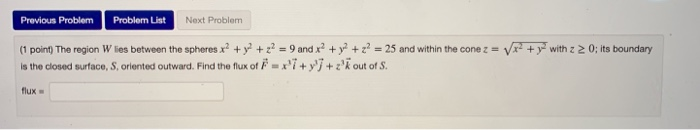Previous Problem Problem List Next Problem x2 + y2 with 2 2 0; its boundary (1 point) The region W lies between the spheres x2 + y2 + z = 9 and x2 + y2 + z = 25 and within the cone z = Is the closed surface, S., oriented outward. Find the flux of F = x +y +z'k out of S. flux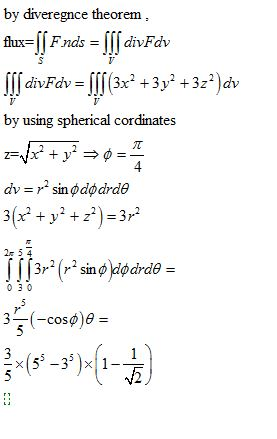#### Earn Coin

Coins can be redeemed for fabulous gifts.

Similar Homework Help Questions
• ### x2 + y2 with z 2 0; its (1 point) The region W lies between the...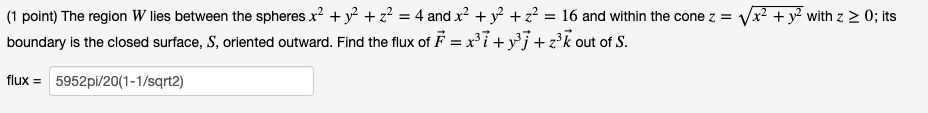x2 + y2 with z 2 0; its (1 point) The region W lies between the spheres x² + y2 + z2 = 4 and x² + y2 + z = 16 and within the cone z = boundary is the closed surface, S, oriented outward. Find the flux of Ě = x; i+y?1+z2k out of S. flux = 5952pi/20(1-1/sqrt2)

• ### 22 + y2 with (1 point) The region W lies between the spheres x2 + y2...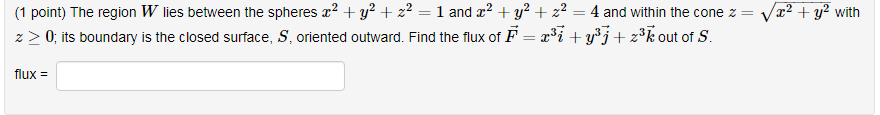22 + y2 with (1 point) The region W lies between the spheres x2 + y2 + z2 = 1 and 22 + y2 + x2 = 4 and within the cone z = z > 0; its boundary is the closed surface, S, oriented outward. Find the flux of F=ri+y +z3k Out of S. flux =

• ### (5) (20 points total) The region W lies between the spheres x2y z2 1 and x2y2 + z2 9 and within t...i found 8pi(2-sqrt(2)) (5) (20 points total) The region W lies between the spheres x2y z2 1 and x2y2 + z2 9 and within the cone z22 +y2with z 2 0; its boundary is the closed surface, S, oriented outward. For G-: < x, y, z >, where -A2 + y2 + z2 . Use the divergence Theorem to computeJI, .ds (5) (20 points total) The region W lies between the spheres x2y z2 1 and x2y2 + z2 9...

• ### < webwork/mast_219_winter_2020 / assignment_10/10 Assignment 10: Problem 10 Previous Problem List Next (1 point) Suppose W...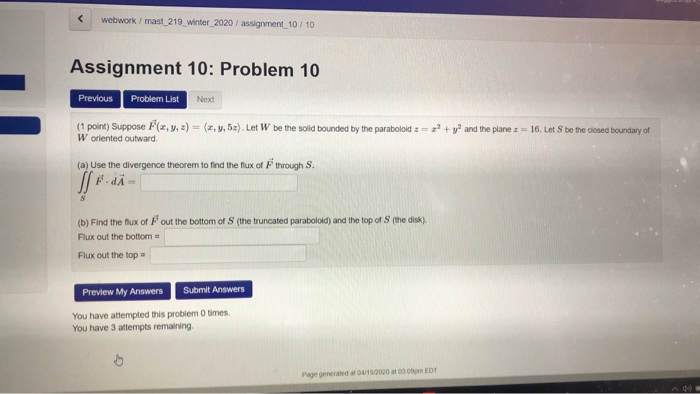< webwork/mast_219_winter_2020 / assignment_10/10 Assignment 10: Problem 10 Previous Problem List Next (1 point) Suppose W oriented outward , y, z) = (x, y, 52). Let W be the solid bounded by the paraboloid := + y and the plane z = 16. Let S be the closed boundary of (a) Use the divergence theorem to find the flux of through S. di- the truncated parabolold) and the top of the disk) (b) Find the flux of Fout the bottom...

• ### 17.2 Stokes Theorem: Problem 2 Previous Problem Problem List Next Problem (1 point) Verify Stokes' Theorem...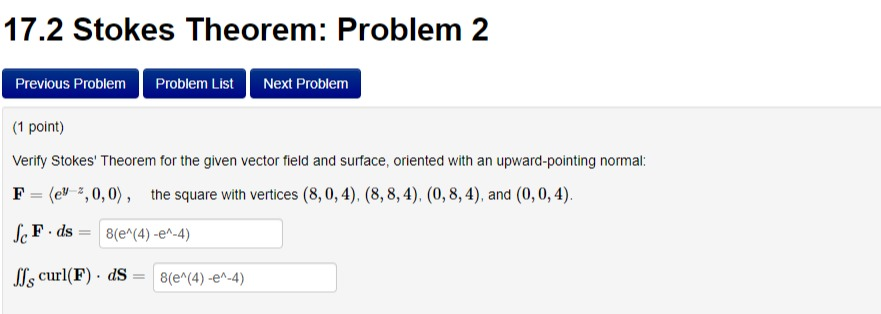17.2 Stokes Theorem: Problem 2 Previous Problem Problem List Next Problem (1 point) Verify Stokes' Theorem for the given vector field and surface, oriented with an upward-pointing normal: F (ell,0,0), the square with vertices (8,0, 4), (8,8,4),(0,8,4), and (0,0,4). ScFids 8(e^(4) -en-4) SIs curl(F). ds 8(e^(4) -e^-4) 17.2 Stokes Theorem: Problem 1 Previous Problem Problem List Next Problem (1 point) Let F =< 2xy, x, y+z > Compute the flux of curl(F) through the surface z = 61 upward-pointing normal....

• ### (1 point) Suppose F(x, y, z) = (x, y, 4z). Let W be the solid bounded...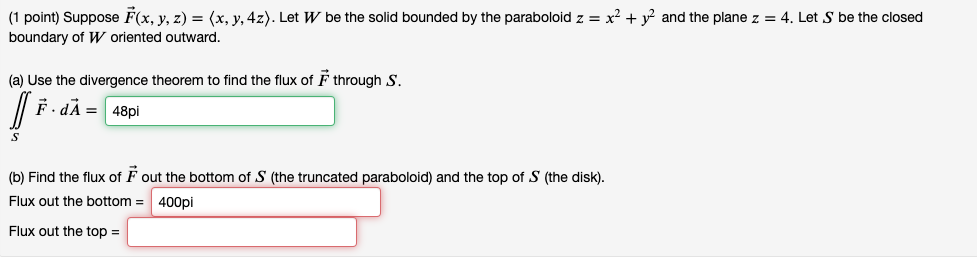(1 point) Suppose F(x, y, z) = (x, y, 4z). Let W be the solid bounded by the paraboloid z = x2 + y2 and the plane z = 4. Let S be the closed boundary of W oriented outward. (a) Use the divergence theorem to find the flux of F through S. ſ FdA = 48pi S (b) Find the flux of F out the bottom of S (the truncated paraboloid) and the top of S (the disk). Flux...

• ### Homework 1: Problem 33 Previous Problem Problem List Next Problem Results for this submission Entered Answer...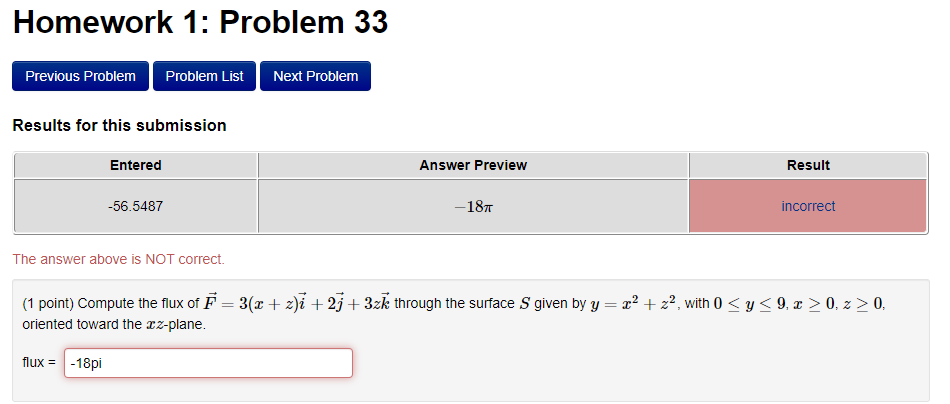Homework 1: Problem 33 Previous Problem Problem List Next Problem Results for this submission Entered Answer Preview Result -56.5487 -187 incorrect The answer above is NOT correct. (1 point) Compute the flux of F = 3(2 + z)i + 25 + 3zk through the surface S given by y = 22 + z2, with 0 <y<9, x > 0, 2 > 0, oriented toward the xz-plane. flux = -18pi

• ### Homework3: Problem 13 Previous ProblemProblem List Next Problem (1 point) Compute the flux of F24zk over the quarte...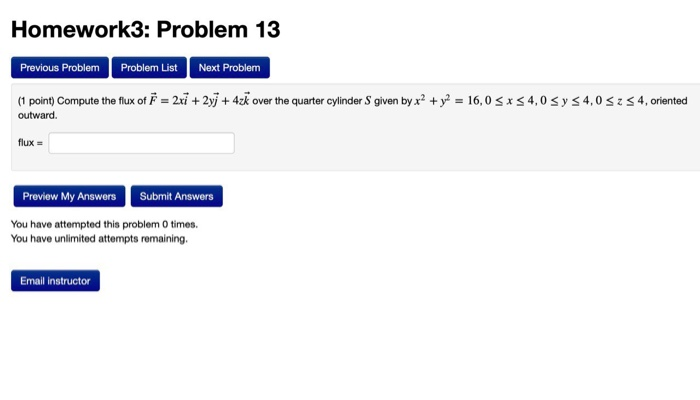Homework3: Problem 13 Previous ProblemProblem List Next Problem (1 point) Compute the flux of F24zk over the quarter cylinder S given byx2y 16,0 s S4,0 s y s4,0S S4, oriented outward. flux- Preview My AnswersSubmit Answers You have attempted this problem O times You have unlimited attempts remaining. Email instructor Homework3: Problem 13 Previous ProblemProblem List Next Problem (1 point) Compute the flux of F24zk over the quarter cylinder S given byx2y 16,0 s S4,0 s y s4,0S S4, oriented...

• ### please just the final answer for both Evaluate the surface Integral || 5. ds for the...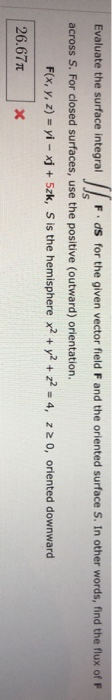please just the final answer for both Evaluate the surface Integral || 5. ds for the given vector fleld F and the oriented surface S. In other words, find the flux of F across S. For closed surfaces, use the positive (outward) orientation. F(x, y, z) = yi - xj + Szk, S is the hemisphere x2 + y2 + y2 = 4, 220, oriented downward 26.677 X Evaluate the surface integral llo F.ds for the given vector field F...

• ### Evaluate the surface integral F dS for the given vector field F and the oriented surface S. In other words, find the flux of F across S. For closed surfaces, use the positive (outward) orientation. F...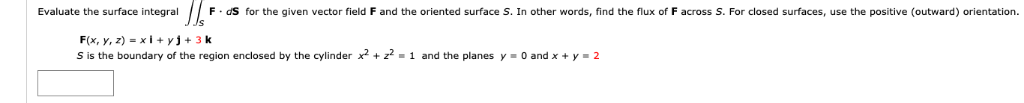Evaluate the surface integral F dS for the given vector field F and the oriented surface S. In other words, find the flux of F across S. For closed surfaces, use the positive (outward) orientation. F(x, y, z) -xi yj+3 k S is the boundary of the region enclosed by the cylinder x2 + z2-1 and the planes y 0 and x y 2 Evaluate the surface integral F dS for the given vector field F and the oriented surface...